# Differential Manometer

Also found in: Medical.

## differential manometer

[‚dif·ə′ren·chəl mə′näm·əd·ər]
(engineering)
An instrument in which the difference in pressure between two sources is determined from the vertical distance between the surfaces of a liquid in two legs of an erect or inverted U-shaped tube when each of the legs is connected to one of the sources.
McGraw-Hill Dictionary of Scientific & Technical Terms, 6E, Copyright © 2003 by The McGraw-Hill Companies, Inc.
The following article is from The Great Soviet Encyclopedia (1979). It might be outdated or ideologically biased.

## Differential Manometer

an instrument for the determination of pressure differences (gradients); it is also used to determine from pressure gradients the levels of liquids and the flow rates of liquids, vapors, or gases. According to the principle of operation, a distinction is made between the liquid type, in which the pressure or vacuum being measured is balanced by a column of liquid, and the mechanical type, in which the pressure is balanced by the elastic forces of various sensing elements, such as membranes, springs, or bellows. The elastic deformation of the sensing element is proportional to the pressure being measured.

Liquid type. Liquid differential manometers are divided into tube, ring, float, and bell types. Tube differential manometers may have two tubes (U-shaped) or one tube (with a vessel and a vertical tube or a vessel and an inclined tube, which serves to increase the precision of the readings when small quantities are being measured). The operation of double-column differential manometers (Figure 1) is based on the use of communicating vessels filled with a column of liquid that is simultaneously a hydraulic lock and generates the hydrostatic pressure that counteracts the pressure being measured. One end of the U-shaped tube, which is filled with a liquid, is connected to a closed volume in which the residual pressure must be determined, and the other end remains open (under barometric pressure). The difference between the liquid levels in the tubes indicates the excess pressure pexc = pabspbar = p; p = hpg, where h is the difference between the liquid levels, ρ is the density of the liquid filling the tubes, and g is the acceleration of free fall. The equation for the single-column differential manometer with a vessel and a vertical tube (Figure 2) is analogous to the equation for the double-column type. The magnitude of displacement of the liquid contained in the tube of the single-column manometer is directly proportional to the pressure gradient being measured and depends on the ratio of the squares of the diameters or areas of the cross sections of the tube and the vessel: f/F = d2/D2. To simplify the measurements, a ratio is adopted for which h2 and h1 will differ by no more than 1 percent; therefore, the quantity h1 may be neglected, and the reading is taken only in terms of the liquid level in the tube. To eliminate error, the scale is made with divisions equal to 0.9 mm rather than 1 mm. The range of measurements using a U-shaped differential manometer is up to 93 kilonewtons per square meter (kN/m2), or 700 mm of mercury (mm Hg), when the pressure of the medium is up to 15 meganewtons per sq m (MN/m2), or 150 kilograms-force per sq cm (kgf/cm2). The accuracy of measurement in the two tubes is ± 1 mm.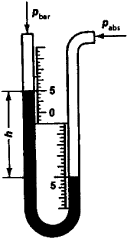Figure 1. Double-column U-shaped differential manometer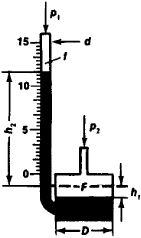Figure 2. Differential manometer with reservoir and vertical tube

The principle of operation of a float-type differential manometer is analogous to that of the single-column differential manometer with a vessel and a vertical tube, with the difference that measurements are made by a float, which transmits changes in the liquid level to a gauge indicator. The range of measurements is 0-133 kN/m2 (0-1,000 mm Hg) when the pressure of the medium is up to 16 MN/m2 (160 kgf/cm2).

The ring differential manometer, or “ring balance,” contains a sensitive element in the form of a hollow ring with a partition (Figure 3). A compensating weight is attached to the lower part of the ring, which is filled with a liquid (water, oil, or mercury). For p1 = p2, the liquid level in both sections of the ring is the same and the center of gravity of the weight is located on the vertical axis, which passes through the center of the ring. For p1 > p2, the liquid level in the left-hand part is lowered, and the liquid level in the right-hand part is raised. The force created by the action of the pressure difference on the partition generates a moment that tends to turn the ring clockwise. The range of measurements of pressure gradients is as follows: at low pressures (when the ring is filled with water), up to 1.6 kN/m2 (160 kgf/m2) when the pressure of the medium is up to 150 kN/m2 (15,000 kgf/m2), and at medium pressures (when the ring is filled with mercury), up to 33 kN/m2 (250 mm Hg) when the pressure of the medium is up to 3.2 MN/m2 (32 kgf/cm2). The basic relative error is ±0.5-1.5 percent.

The bell differential manometer (Figure 4) consists of a bell that is immersed in a liquid and that moves under the influence of the pressure difference between the interior (greater) and the exterior (smaller) of the bell. The force counteracting the pressure being measured is generated by weighting the bell (hydrostatic equilibration) or by the deformation of a spring by which the bell is suspended (mechanical equilibration). The range of measurements of the pressure gradient is 40 N/m2-4 kN/m2 (4-400 kgf/m2) when the pressure of the medium is 10 kN/m2-0.3 MN/m2 (1,000 kgf/m2-3 kgf/cm2).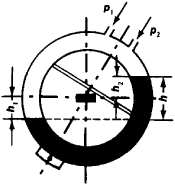Figure 3. Diagram of a ring differential manometer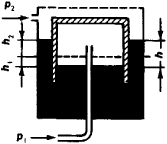Figure 4. Diagram of a bell differential manometer

Mechanical type. Mechanical differential manometers are divided into the membrane type, with a flat elastic metal membrane (Figure 5) or a nonmetallic membrane, and the bellows type. In the membrane type, the elastic metallic membrane bends under the influence of the pressure being measured, and the magnitude of the bending is used to determine the pressure. In some designs, the membrane serves only to separate the chambers. The counteracting force upon deformation is generated by a calibrated cylindrical spiral spring, which takes the load from the membrane. Some membrane differential manometers contain protection from a one-sided overload and may be used for measuring not only gradients but also excess pressures. The measurement range is 0-6.3 kN/m2 (0-630 kgf/m2) and 0.16-0.63 MN/m2 (1.6-6.3 kgf/cm2); pressure gradients up to 133 kN/m2 (1,000 mm Hg) may be measured, when the maximum pressure of the medium is up to 60 MN/m2 (600 kgf/cm2). The basic relative error is ±1.5 percent. Differential manometers with non-metallic membranes (rubber and other materials) contain only a cylindrical spiral spring, do not respond to bending moments and compression forces, and operate only in the extension mode. To increase the displacement, they are made in corrugated form and have a rigid center consisting of two metal disks. The range of measurements of pressure gradients is up to 133 kN/m2 (1,000 mm Hg) when the pressure of the medium is up to 6.4 MN/m2 (64 kgf/cm2). The basic relative error is ±1-2 percent.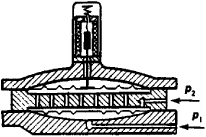Figure 5. Differential manometer with an elastic membrane and electrical transmission of readings

The sensitive element of bellows differential manometers consists of a corrugated metal housing (bellows) with a calibrated cylindrical spiral spring. The bellows divides the cavity of the manometer into two chambers. The higher pressure is introduced into the space above the bellows, and the lower pressure is introduced into the space inside it. Under the influence of the pressure difference, the bellows bends to a degree proportional to the pressure being measured. The range of measurements is up to 25 kN/m2 (2,500 kgf/m2) when the pressure of the medium is up to 32 MN/m2 (320 kgf/cm2). The basic relative error is ±0.5-1 percent.

The float, ring, bell, and mechanical differential manometers are manufactured as indicating, recording, and scaleless instruments (with electrical or pneumatic transmission of readings). Differential manometers for the determination of flow rates by the variable gradient method are produced with integrators and adders. Further development of differential manometer design is aimed at perfecting the mechanical differential manometers.